## Tamilnadu Samacheer Kalvi 11th Maths Solutions Chapter 4 Combinatorics and Mathematical Induction Ex 4.2

11th Maths Exercise 4.2 Question 1.
If (n – 1) P3 : nP4, find n:
Solution: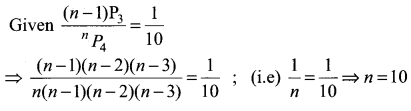Class 11 Maths Ex 4.2 Solutions Question 2.
If 10Pr – 1 = 2 × 6Pr, find r.
Solution: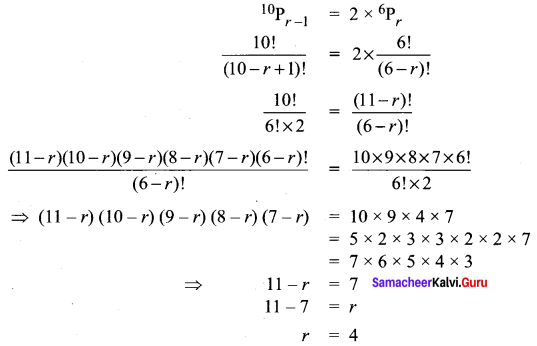11th Maths Chapter 4 Exercise 4.2 Question 3.

(i) Suppose 8 people enter an event in a swimming meet. In how many ways could the gold, silver and bronze prizes be awarded?
Solution:
From 8 persons we have to select and arrange 3 which can be done in 8P3 ways So the prizes can be awarded in 8P3 = 8 × 7 × 6 = 336 ways

(ii) Three men have 4 coats, 5 waist coats and 6 caps. In how many ways can they wear them?
Solution:
Selecting and arranging 3 coats from 4 can be done in 4P3 ways
Selecting and arranging 3 waist coats from 5 can be done in 5P3 ways Selecting and arranging 3 caps from 6 can be done in 6P3 ways
∴ Total number of ways = 4P3 × 5P3 × 6P3 = 172800 ways

Combinatorics And Mathematical Induction Question 4.
Determine the number of permutations of the letters of the word SIMPLE if all are taken at a time?
Solution:
SIMPLE
Total Number of letters = 6
They can be arranged in 6! ways
∴ Number of words = 6!
= 6 × 5 × 4 × 3 × 2 × 1 = 720

Ex 4.2 Class 11 Maths Question 5.
A test consists of 10 multiple choice questions. In how many ways can the test be answered if

(i) Each question has four choices?
Solution:
Each question has 4 choices. So each questions can be answered in 4 ways.
Number of Questions = 10
So they can be answered in 410 ways

(ii) The first four questions have three choices and the remaining have five choices?
Solution:
The first four questions have 3 choices. So they can be answered in 34 ways. Remaining 6 questions have 5 choices. So they can be answered in 56 ways.
So all 10 questions can be answered in 34 × 56 ways.

(iii) Question number n has n + 1 choices?
Solution:
Given question n has n + 1 choices
question 1 has 1 + 1 = 2 choices
question 2 has 2 + 1 = 3 choices
question 3 has 3 + 1 = 4 choices
question 4 has 4 + 1 = 5 choices
question 5 has 5 + 1 = 6 choices
question 6 has 6 + 1 = 7 choices
question 7 has 7 + 1 = 8 choices
question 8 has 8 + 1 = 9 choices
question 9 has 9 + 1 = 10 choices
So the number of ways of answering all the 10 questions
= 2 × 3 × 4 ×…. × 11 = 11! ways

Exercise 4.2 Class 11 Question 6.
A student appears in an objective test which contain 5 multiple choice questions. Each question has four choices out of which one correct answer.

(i) What is the maximum number of different answers can the students give?
Solution:
Selecting a correct answer from the 4 answers can be done in 4 ways.
Total number of questions = 5 So they can be answered in 45 ways

(ii) How will the answer change if each question may have more than one correct answers?
Solution:
When each question has more than 1 correct answer. Selecting the correct choice from the 4 choice can be done is 4C1 or 4C2 or 4C3 or 4C4 ways.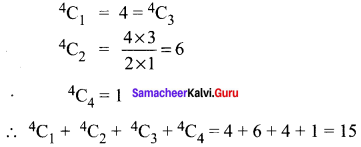Each question can be answered in 15 ways.
Number of questions = 5
∴ Total number of ways = 155

Class 11 Maths Chapter 4 Exercise 4.2 Solution Question 7.
How many strings can be formed from the letters of the word ARTICLE, so that vowels occupy the even places?
Solution:
ARTICLE
Vowels A, I, E = 3
Total number of places = 7
1 2 3 4 5 6 7
Number of even places = 3
3 Vowels can occupy 3 places in 3! = 3 × 2 × 1 = 6 ways
Then the remaining 4 letters can be arranged in 4! ways
So total number of arrangement = 3! × 4! = 6 × 24 = 144 ways

Exercise 4.2 Maths Class 11 Solutions Question 8.
8 women and 6 men are standing in a line.

(i) How many arrangements are possible if any individual can stand in any position?
Solution:
Total number of persons = 8 + 6 = 14
They can be arranged in 14! ways

(ii) In how many arrangements will all 6 men be standing next to one another?
Solution:
There are 6 men and 8 women. To make all 6 men together treat them as 1 unit. Now there are 1 + 8 = 9 persons.
They can be arranged in 9! ways. After this arrangement the 6 men can be arranged in 6! ways. So total number of arrangement = 9! × 6!

(iii) In how many arrangements will no two men be standing next to one another?
Solution:
Since no two men be together they have to be placed between 8 women and before and after the women.
w | w | w | w | w | w | w | w
There are 9 places so the 6 men can be arranged in the 9 places in 9P6 ways.
After this arrangement, the 8 women can be arranged in 8! ways.
∴ Total number of arrangements = (9P6) × 8!

Ex 4.2 Class 11 Question 9.
Find the distinct permutations of the letters of the word MISSISSIPPI?
Solution:
MISSISSIPPI
Number of letters = 11
Here M – 1 time
I – 4 times
S – 4 times
P – 2 times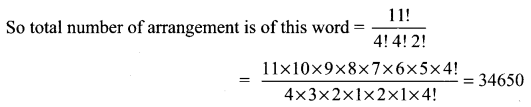4.2 Maths Class 11 Question 10.
How many ways can the product a2b3c4 be expressed without exponents?
Solution:
a2b3c4 = aabbbcccc
Number of letters = 9
a = 2 times,
b = 3 times,
c = 4 times,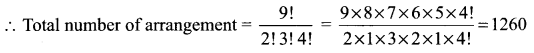Class 11 Maths Chapter 4 Exercise 4.2 Question 11.
In how many ways 4 mathematics books, 3 physics books, 2 chemistry books and 1 biology book can be arranged on a shelf so that all books of the same subjects are together.
Solution:
Number of maths book = 4
Number of physics books = 3
Number of chemistry books = 2
Number of biology books = 1
Since we want books of the same subjects together, we have to treat all maths books as 1 unit, all physics books as 1 unit, all chemistry books as 1 unit and all biology books as 1 unit. Now total number of units = 4
They can be arranged in 4! ways. After this arrangement.
4 maths book can be arranged in 4! ways
3 physics book can be arranged in 3! ways
2 chemistry book can be arranged in 2! ways and 1 biology book can be arranged in 1! way
∴ Total Number of arrangements 4! 4! 3! 2! = 6912

11th Maths 4th Chapter Solutions Question 12.
In how many ways can the letters of the word SUCCESS be arranged so that all Ss are together?
Solution:
SUCCESS
Number of letters = 7
Number of ‘S’ = 3
Since we want all ‘S’ together treat all 3 S’s as 1 unit.
Now the remaining letters = 4
∴ Total number of unit = 5
They can be arranged in 5! ways of them C repeats two times.
So total number of arrangements = $$\frac{5 !}{2 !}$$ = 60

Exercise 4.2 Class 11 Maths Question 13.
A coin is tossed 8 times,

(i) How many different sequences of heads and tails are possible?
Solution:
Number of coins tossed = 8
Number of out come for each toss = 2
Total number of out comes = 28

(ii) How many different sequences containing six heads and two tails are possible?
Solution:
Getting 6 heads and 2 tails can be done in 8P6 or 8P2 ways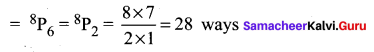10th Maths Exercise 4.2 11th Sum Question 14.
How many strings are there using the letters of the word INTERMEDIATE, if

(i) The vowels and consonants are alternative
Solution:
INTERMEDIATE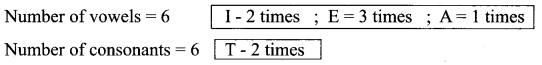The number of ways in which vowels and consonants are alternative = $$\frac{6 ! 6 !}{3 ! 2 !}=$$ 43200

(ii) All the vowels are together
Solution:
The number of arrangements:
Keeping all the vowels as a single unit. Now we have 6 + 1 = 7 units which can be arranged in 7! ways.
Now the 6 consonants can be arranged in $$\frac{6 !}{2 !}$$ (T occurs twice) ways
in vowels I – repeats thrice
and E – repeats twice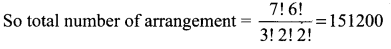(iii) Vowels are never together (and) (iv) No two vowels are together.
Solution:
Vowels should not be together = No. of all arrangements – No. of all vowels together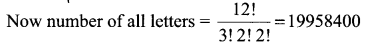So number of ways in which No two vowels are together = 19958400 – Number of ways in which vowels are together = 19958400 – 151200 = 19807200

Tn Class 11 Maths Solutions Question 15.
Each of the digits 1,1, 2, 3, 3 and 4 is written on a separate card. The seven cards are then laid out in a row to form a 6-digit number.

(i) How many distinct 6-digit numbers are there?
Solution:
The given digits are 1, 1, 2, 3, 3, 4
The 6 digits can be arranged in 6! ways
In which 1 and 3 are repeated twice.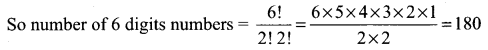(ii) How many of these 6-digit numbers are even?
Solution:
To find the number even numbers
The digit in unit place is 2 or 4 which can be filled in 2 ways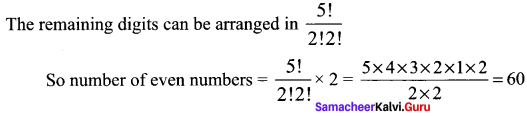(iii) How many of these 6-digit numbers are divisible by 4?
Solution:
To get a number -f- by 4 the last 2 digits should be -r- by 4 So the last two digits will be 12 or 24 or 32.
When the last 2 digits are 1 and 2.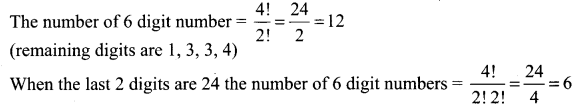When the last 2 digit are 3 and number of 6 digit numbers (remaining number 1, 1, 3, 4)
So there of 6 digit numbers ÷ by 4 = 12 + 6 + 12 = 30

Exercise 4.2 Class 11 Pdf Question 16.
If the letters of the word GARDEN are permuted in all possible ways and the strings thus formed are arranged in the dictionary order, then find the ranks of the words
(i) GARDEN
(ii) DANGER.
Solution:
The given letters are GARDEN.
To find the rank of GARDEN:
The given letters in alphabetical order are A D E G N R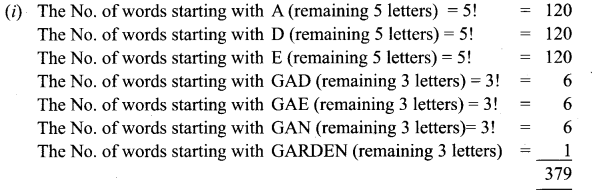The rank of GARDEN is 379
To find the rank of DANGER

(ii) The No. of words starting with A = 5! =120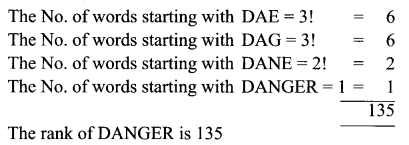Samacheer Kalvi 11th Maths Answers Question 17.
Find the number of strings that can be made using all letters of the word THING. If these words are written as in a dictionary, what will be the 85th string?
Solution:
(i) Number of words formed = 5! = 120
(ii) The given word is THING
Taking the letters in alphabetical order G H I N T
To find the 85th word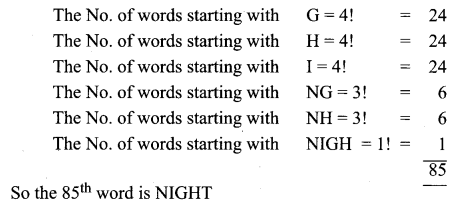Question 18.
If the letters of the word FUNNY are permuted in all possible ways and the strings thus formed are arranged in the dictionary order, find the rank of the word FUNNY.
Solution:
The given word is FUNNY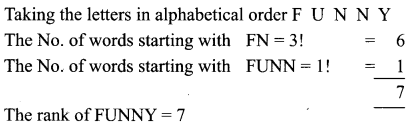Question 19.
Find the sum of all 4-digit numbers that can be formed using digits 1, 2, 3, 4, and 5 repetitions not allowed?
Solution:
The given digits are 1, 2, 3, 4, 5
The no. of 4 digit numbers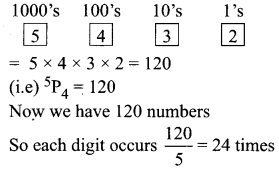Sum of the digits = 1 + 2 + 3 + 4 + 5 = 15
Sum of number’s in each place = 24 × 15 = 360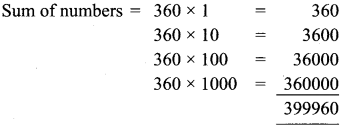Question 20.
Find the sum of all 4-digit numbers that can be formed using digits 0, 2, 5, 7, 8 without repetition?
Solution:
The given digits are 0, 2, 5, 7, 8
To get the number of 4 digit numbers
1000’s place can be filled in 4 ways (excluding 0)
100’s place can be filled in 4 ways (excluding one number and including 0)
10’s place can be filled in (4 – 1) = 3 ways
and unit place can be filled in (3 – 1) = 2 ways
So the number of 4 digit numbers = 4 × 4 × 3 × 2 = 96

To find the sum of 96 numbers:

In 1000’s place we have the digits 2, 5, 7, 8. So each number occurs $$\frac{96}{4}$$ = 24 times.
Now in 100’s place 0 come 24 times. So the remaining digits 2, 5, 7, 8 occurs 96 – 24 = $$\frac{72}{4}$$ = 18 times
Similarly in 10’s place and in unit place 0 occurs 24 times and the remaining digits 2, 5, 7, 8 occurs 18 times.
Now sum of the digits = 2 + 5 + 7 + 8 = 22
Sum in 1000’s place = 22 × 24 = 528
Sum in 100’s, 10’s and in unit place = 22 × 18 = 396
∴ Sum of the 4 digit numbers is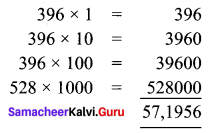### Samacheer Kalvi 11th Maths Solutions Chapter 4 Combinatorics and Mathematical Induction Ex 4.2 Additional Questions

Question 1.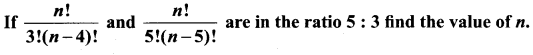Solution: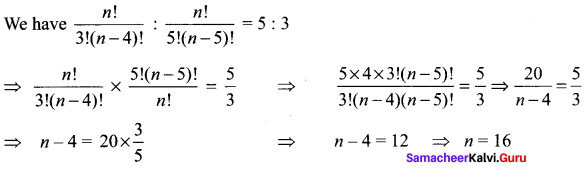Question 2.
How many 3-digit even numbers can be made using the digits 1, 2, 3, 4, 6, 7 if no digit is repeated?
Solution:
Here total number of digits = 6
The unit place can be filled with any one of the digits 2, 4, 6.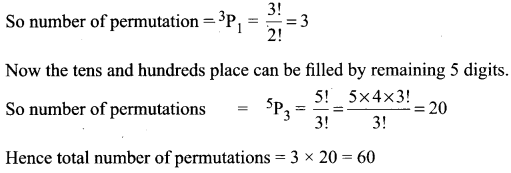Question 3.
Find n if n – 1P3 : nP4 = 1 : 9
Solution: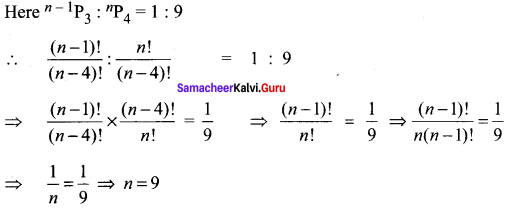Question 4.
How many words can be formed by using the letters of the word ORIENTAL so that A and E always occupy the odd places?
Solution:
[Hint: There are 4 odd places in the word]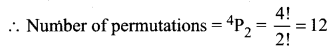Now the remaining 6 places filled with remaining 6 letters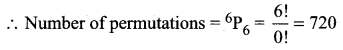Hence the total number of permutations = 12 × 720 = 8640

Question 5.
Out of 18 points in a plane, no three are in the same line except five points which are collinear. Find the number of lines that can be formed joining the points.
Solution:
Total number of points = 18
Out of 18 numbers, 5 are collinear and we get a straight line by joining any two points.
∴ Total number of straight line formed by joining 2 points out of 18 points = 18C2
Number of straight lines formed by joining 2 points out of 5 points = 5C2
But 5 points are collinear and we get only one line when they are joined pairwise.
So, the required number of straight lines are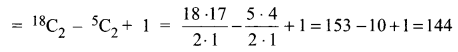Hence, the total number of straight lines = 144

Question 6.
We wish to select 6 person from 8 but, if the person A is choosen, then B must be choosen. In how many ways can selections be made?
Solution:
Total number of persons = 8
Number of persons to be selected = 6
Condition is that if A is choosen, B must be choosen

Case I: When A is choosen, B must be choosen
Number of ways = 6C4
[∴ A and B are set to be choosen]

Case II: When A is not choosen, then B may be choosen
∴ Number of ways = 7C6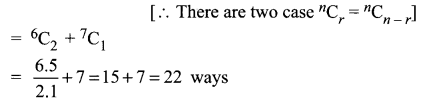Hence, the required number of ways = 22

Question 7.
How many 3-digit even numbers can be made using the digits 1, 2, 3, 4, 6, 7, if no digit is repeated?
Solution:
For 3-digit even numbers, the unit’s place can be occupied by one of the 3 digits 2, 4 or 6. The remaining 5 digits can be arranged in the remaining 2 places in 5P2 ways.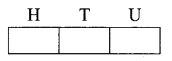∴ By the multiplication rule, the required number of 3-digit even numbers is 3 × 5P2 = 3 × 5 × 4 = 60.

Question 8.
Find the number of 4-digit numbers that can be formed using the digits 1, 2, 3, 4, 5 if no digit is repeated. How many of these will be even?
Solution:
For 4 digit numbers, we have to arrange the given 5 digits in 4 vacant places. This can be done in 5P4 = 5 × 4 × 3 × 2 = 120 ways.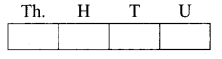For 4-digit even numbers, the unit’s place can be occupied by one of the 2 digits 2 or 4. The remaining 4 digits can be arranged in the remaining 3 places in 4P3 ways.
∴ By the multiplication rule, the required number of 4-digit even numbers is 2 × 4P3 = 2 × 4 × 3 × 2 = 48.

Question 9.
Find r if

(i) 5Pr = 2 6Pr – 1 4
Solution: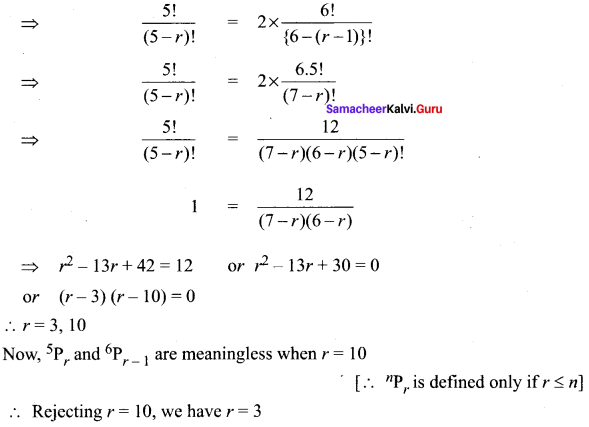(ii) 5Pr = 6Pr – 1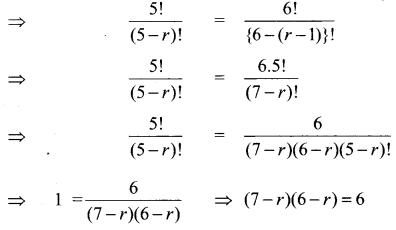⇒ 42 – 13r + r2 = 6 ⇒ r2 – 13r + 36 = 0
⇒ (r – 4)(r – 9) = 0 ⇒ r = 4, 9
Now, we know that nPr is meaningful only when r ≤ n.
5Pr and 6Pr – 1 are meaningless when r ≤ 9.
∴ Rejecting r = 9, we have r = 4

Question 10.
How many words, with or without meaning, can be made from the letters of the word MONDAY, assuming that no letter is repeated, if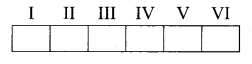(i) 4 letters are used at a time
(ii) all letters are used at a time
(iii) all letters are used but first letter is a vowel?
Solution:
The word MONDAY has 6 distinct letters.

(i) 4 letters out of 6 can be arranged in 6P4 ways.
∴ The required number of words = 6P4 = 6 × 5 × 4 × 3 = 360

(ii) 6 letters can be arranged among themselves in 6P6 ways.
∴ The required number of words = 6P6 = 6!
= 1 × 2 × 3 × 4 × 5 × 6 = 720.

(iii) The first place can be filled by anyone Of the two vowels O or A in 2 ways. The remaining 5 letters can be arranged in the remaining 5 places II to VI in 5P5 = 5! ways.
∴ By the multiplication rule, the required number of words = 2 × 5! = 2 × 1 × 2 × 3 × 4 × 5 = 240